Courses

Olympiad Test: Profit And Loss - 2

10 Questions MCQ Test Mathematical Olympiad Class 8 | Olympiad Test: Profit And Loss - 2

Description
Attempt Olympiad Test: Profit And Loss - 2 | 10 questions in 20 minutes | Mock test for Class 8 preparation | Free important questions MCQ to study Mathematical Olympiad Class 8 for Class 8 Exam | Download free PDF with solutions
QUESTION: 1

The cost of a mobile phone is Rs. 3000. A gain of 10% should be made after a discount of 20%. What is the marked price of the mobile phone?

Solution:

Selling Price = 3000 × 11/1000 = 3300
Let Marked Price be ` x
x - 20% of x = x -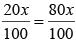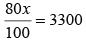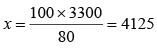QUESTION: 2

Rajesh has to pay 6% sales tax in addition to the price of a certain article. What is the price if he pays Rs. 53 to buy the article?

Solution:

Let the price be Rs. x.
then x + 6% of x = 53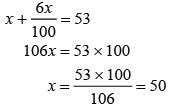QUESTION: 3

Bunti buys a leather coat costing Rs. 900 at Rs. 999. After paying the sales tax. What is rate of sales tax charged on the coat?

Solution:

Let sales tax = x %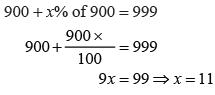QUESTION: 4

A shopkeeper increases the price of an item by 10% and then allows a discount of 15%. How much has the customer to pay if the item was initially prieod at Rs. 1200 ?

Solution:

Price = Rs. 1200
Increased Price = 1200 + 10% of 1200
= 1200 + 120 = 1320
Discount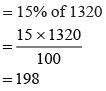The net payable amount = 1320 – 198
= Rs.1122

QUESTION: 5

What amount that Jay will receive if he deposits Rs. 8000 for 3 years at 10% per annum compounded annually?

Solution:

Here A =P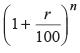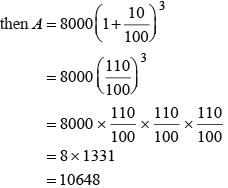QUESTION: 6

A businessman marks his good at 40% above the cost price and allows a discount of 25%. What is his gain percent?

Solution:

Let the cost price be Rs. 100
Marked price = Rs. 140
Discount = 25% of 140 =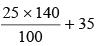Selling price = 140 – 35 = 105
Gain Percent = 105 – 100 = 5%

QUESTION: 7

The cost price of 12 books is equal to selling price of 15 books. What is the loss percent?

Solution:

Let the cost price of 12 books = ₹ x.
Cost price of 1 book = x/12
Selling price of 15 books = ₹ x.
S.P. of 1 book = x/15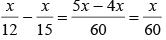Loss percent = x / 60 x / 12 x 100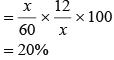QUESTION: 8

Calculate the compound interest on Rs. 10000 at 10% per annum for 3 years, if interest compounded annually?

Solution:

Here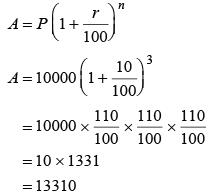∴ C.I. = A P = 13310 - 10000
= Rs. 3310

QUESTION: 9

The value of a machine at the rate of 20% per annum. It was purchased 2 years ago if its present value is Rs. 4000, for how much was it purchased?

Solution:

Let the purchase price = ` x
then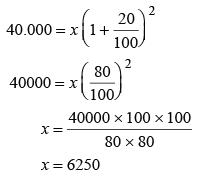QUESTION: 10

If the simple interest on a sum of money at 5% per annum for 3 years is Rs. 1200. Then what is the compound interest on the same sum for the same period at the same rate?

Solution:

Let sum = Rs. P
then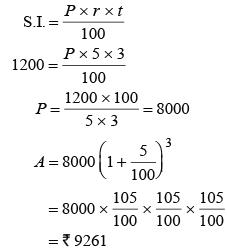∴ C.I. =  9261 - 8000 = 1261Use Code STAYHOME200 and get INR 200 additional OFF Use Coupon Code

Track your progress, build streaks, highlight & save important lessons and more!

Similar Content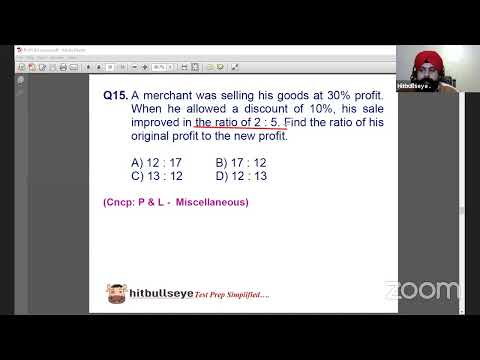Related tests